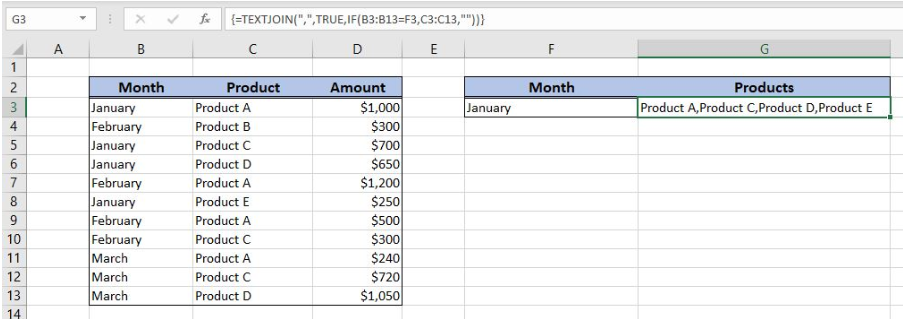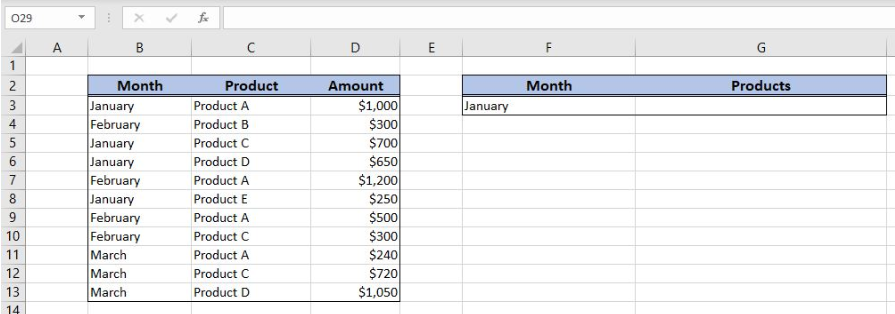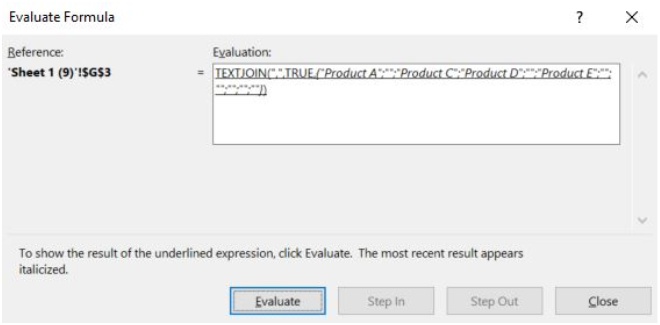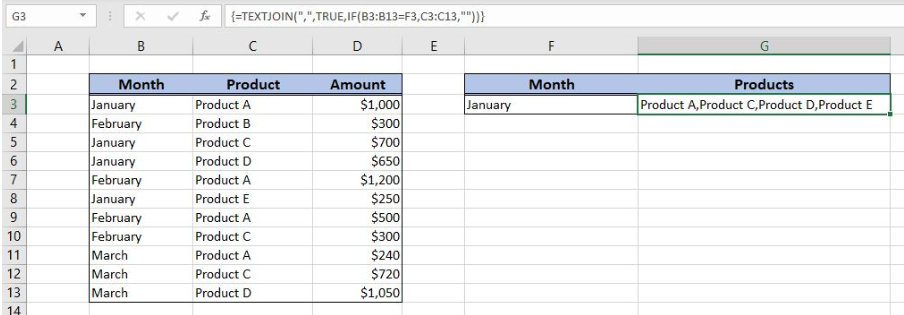Get instant live expert help with Excel or Google Sheets“My Excelchat expert helped me in less than 20 minutes, saving me what would have been 5 hours of work!”

#### Post your problem and you’ll get expert help in seconds.

Your message must be at least 40 characters
Our professional experts are available now. Your privacy is guaranteed.

# Learn How to Return Multiple Match Results in Excel

Excel allows us to return multiple match result of a lookup using the TEXTJOIN and IF functions. This step by step tutorial will assist all levels of Excel users to learn how to return multiple match results in Excel.Figure 1. The final result of the formula

## Syntax of the TEXTJOIN Formula

The generic formula for the TEXTJOIN function is:

`=TEXTJOIN(delimiter, ignore_empty, text1, [text2], ...)`

The parameters of the TEXTJOIN function are:

• delimiter – a string that will be used as a separator between the text items
• ignore_empty – TRUE for ignoring empty cells
• text1, [text2] – text strings that will be joined

## Syntax of the IF Formula

The generic formula for the IF function is:

`=IF(logical_test, value_if_true, value_if_false)`

The parameters of the IF function are:

• logical_test – a logical expression that we want to check
• value_if_true – a value which the function returns if a logical_test is TRUE
• value_if_false – a value which the function returns if a logical_test is FALSE.

## Setting up Our Data for the Example

Our table consists of 3 columns: “Month” (column B), “Product” (column C) and “Amount” (column D). In the cell F3, we have the lookup month, while in the cell G3 we want to get all products from column C with a matching month.Figure 2. Data that we will use in the example

## Return Multiple Match Results of the Lookup Using the TEXTJOIN and IF Functions

In the cell G3, we want to get all matching products from column C, which have a month equal to January.

The formula looks like:

`=TEXTJOIN(",", TRUE, IF(B3:B13=F3, C3:C13, ""))`

The logical_test of the IF function is B3:B13=F3. The value_if_true is C3:C13 and the value_if_false is “”. The text1, [text2] parameters are the results of the IF function. The delimiter is “,“, while the ignore_empty is TRUE.

To apply the formula, we need to follow these steps:

• Select cell E3 and click on it
• Insert the formula: `=TEXTJOIN(",", TRUE, IF(B3:B13=F3, C3:C13, ""))`
• Press Ctrl+Shift+Enter to enter the array function.

If we evaluate the formula, we can see that he result of the IF function is the following array:Figure 3. Evaluate the formulaFigure 4. Using the formula to return multiple match results

The resulting array of the IF function is the text parameter for the TEXTJOIN function. Therefore, the result of the whole formula in G3 is “Product A,Product C,Product D,Product E”.

Most of the time, the problem you will need to solve will be more complex than a simple application of a formula or function. If you want to save hours of research and frustration, try our live Excelchat service! Our Excel Experts are available 24/7 to answer any Excel question you may have. We guarantee a connection within 30 seconds and a customized solution within 20 minutes.

Solution examplesCould you help me by explaining the IF function?
Solved by G. L. in 24 minsI have a question about Conditional Formatting. =IF('Sheet1'!A1="X",IF('Sheet1'!B1="Y", TRUE, FALSE),FALSE) the set the cell background to red. I would like to make it so that if Sheet1'!B1="Z" I set the back to blue. How can I achieve this.
Solved by B. F. in 39 minsI need to find out if the 2018 yearly sales goals were met if the yearly sales were \$25,000 or more using an IF logical function and to set the formula to return a value of YES if met, and NO if not
Solved by A. A. in 18 minsI filtered data in column A (it is labeled ID #) of a data set. On my next worksheet, column A is also the ID #, but it is a different data set. I want to filter the ID #'s the same for the two sheets, but how do I do that being that I am working with two different sets of data?
Solved by V. H. in 22 minsi want to create a very simple if function in a cell which is, if one cell is less than 1250, then the automatic value in the current cell is 250, dead easy, for someone who knows! Thank you.
Solved by A. H. in 14 mins